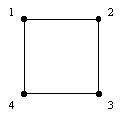## Project 12: Cuboids

Everyone is familiar with the ordinary 3-dimensional cube. One of its features is that it is highly symmetric. In fact, there are 48 different transformations of the cube that preserve the cube. (These transformations include rotations, reflections in lines, and reflections in planes.) In order to understand better the problem to be posed below we will first consider the analog of the problem to be considered for the square.

The square shown labeled in the diagram below has 8 symmetries (isometries) that preserve it. Some of these symmetries are rotations and some of them are reflections.These 8 transformations are referred as the symmetry group of the square. The result of performing one transformation after another yields another transformation. For example, one can rotate by 90 degrees followed by a reflection in the line determined by points 2 and 4.

One way to write down these symmetries is using the notation that appears below, which tells where each original vertex of the square is after being transformed. Think of the labels as being fixed and the points as moving. Record the labels before and after the motion of the points. Here are the eight transformations, the top row shows the labels initially and the bottom row the labels after the transformation is completed:

1234 1234 1234 1234 1234 1234 1234 1234

1234 4123 3412 2341 4321 2143 3214 1432.

This collection of 8 transformations has various subsets of transformations which are subgroups of the original group. For example, the square has a collection of 4 symmetries, its rotations, which form a subgroup of the original group of symmetries. This rotation subgroup consists of:

1234 1234 1234 1234

1234 4123 3412 2341.

It turns out there are various quadrilaterals which have subgroups of the symmetry group of the square as their symmetry groups. For example, the rhombus or the rectangle have four element subgroups as symmetries. However, no quadrilateral has the rotation subgroup of symmetries as its exact set of symmetries. It turns out there are seven types quadrilaterals when looked at from a symmetry point of view. (Examples: rhombus, rectangle, equilateral trapezoid, etc.)

Problems

1. A solid which is structurally like a cube will be called a cuboid. Determine all of the different types of cuboids from a symmetry point of view. (It will probably be helpful to make physical models to understand what is going on.)

2. What mixtures of four sided faces can cuboids have? (Are there cuboids whose faces are all trapezoids and rectangles? All rhombuses and rectangles? All trapezoids?)

3. For each cuboid consider all the nets of the cuboid that are possible. How does the number of nets for the cuboid compare with the number of nets for the cube? (A net of a polyhedron P is a planar pattern of polygons which can be folded up to form P.)

References:

1. Budden, F., The Fascination of Groups, Cambridge U. Press, New York, 1978.

2. Burn, R., Groups: A Path to Geometry, Cambridge U. Press, New York, 1985.

3. Grossman, I. and W. Magnus, Groups and Their Graphs, Mathematical Association of America, Washington, 1964.

Joseph Malkevitch
Department of Mathematics and Computing
York College (CUNY)
Jamaica, New York 11451-0001
email: malkevitch@york.cuny.edu
(Comments and results related to the project above are welcome.)

Acknowledgements
Some of this work was prepared with partial support from the National Science Foundation (Grant Number: DUE 9555401) to the Long Island Consortium for Interconnected Learning (administered by SUNY at Stony Brook, Alan Tucker, Project Director).